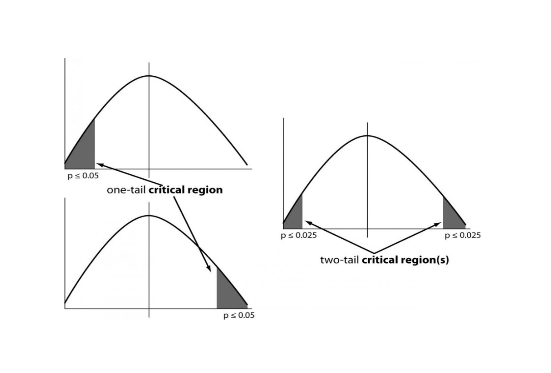# One & Two Tailed Tests

## Summary

• Hypothesis in simpler words is basically a claim that we want to test or investigate
• The ”Null Hypothesis” denoted as${ H }_{ 0 }$, this means testing a claim that already has some established parameters
• The ”Alternative Hypothesis” is denoted as${ H }_{ a }$, this is known as the research hypothesis. It involves the claim to be tested
• One tailed test indicates that the null hypothesis should be rejected when the test value is in the critical region on one side of the mean
• In Two tailed test the null hypothesis should be rejected when the test value is in either of the two critical regions

#### Introduction to Hypothesis

Hypothesis in simpler words is basically a claim that we want to test or investigate. Which means doing sampling and getting the information and then testing the hypothesis. It could just be any idea that we want to test. A few types of Hypothesis are:

• The ”Null Hypothesis” denoted as${ H }_{ 0 }$, this means testing a claim that already has some established parameters. The null hypothesis is always the accepted fact. Some of the examples of null hypotheses that are generally accepted as being true are:
(i) DNA is shaped like a double helix.
(ii) There are 8 planets in the solar system (excluding Pluto).
• The ”Alternative Hypothesis” is denoted as${ H }_{ a }$, this is known as the research hypothesis. It involves the claim to be tested.

Now that you understand what Hypothesis is, let’s move on to study one tailed and two tailed tests.

1. One tailed test consists of two types of tests:

i) Left tailed test: A hypothesis test performed if the parameter is suspected to be less than the assumed parameter of the null hypothesis.
ii) Right tailed test: A hypothesis test performed if the parameter is suspected to be greater than the assumed parameter of the null hypothesis.

For one tailed tests we do either left tailed test or right tailed test. This test indicates that the null hypothesis should be rejected when the test value is in the critical region on one side of the mean. Also remember that in the one-tailed test the value of the parameter can be as high as you like.

2. Two tailed test is a hypothesis test performed if there is no reason to think the parameter is higher or lower than the assumed parameter of the null hypothesis. The null hypothesis should be rejected when the test value is in either of the two critical regions. Two tailed test simply show a difference.

These are shown in the figure below.#### Example #1

Q. A light bulb manufacturer claims that its’ energy saving light bulbs last an average of 60 days. Set up a hypothesis test to check this claim and comment on what sort of test we need to use.

Solution:

As we have:

H0: The mean lifetime of an energy-saving light bulb is 60 days.
H1: The mean lifetime of an energy-saving light bulb is not 60 days.

Because of the “is not” in the alternative hypothesis, we have to consider both the possibility that the lifetime of the energy-saving light bulb is greater than 60 and that it is less than 60. This means we have to use a two-tailed test.

#### Example #2

Q. A premium golf ball production line must produce all of its balls to 1.615 ounces in order to get the top rating (and therefore the top dollar). Samples are drawn hourly and checked. If the production line gets out of sync with a statistical significance of more than 1%, it must be shut down and repaired. This hour’s sample of 18 balls has a mean of 1.611 ounces and a standard deviation of 0.065 ounces. Do you shut down the line?

Solution:

H0: The population mean (µ) = 1.615
HA: The population mean ≠ 1.615 (hence a 2-tailed test)

Since we are doing a two-tailed test, we have to divide the level of significance in half.$\alpha \quad =\quad 0.01$$\frac { \alpha }{ 2 } \quad =\quad 0.005$$\rho \quad =\quad 1\quad -\quad \frac { \alpha }{ 2 }$$\rho \quad =\quad 99.5%$

Now From the tables we can see that${ t }_{ p }\quad =\quad 2.898$

Now the critical regions are > 2.899 and < -2.898

We calculate${ t }_{ c }$ now using the formula:${ t }_{ c }\quad =\quad \frac { Y\quad -\quad \mu }{ { s }/{ \sqrt { n } } }$${ t }_{ c }\quad =\quad \frac { 1.611\quad -\quad 1.615 }{ { 0.065 }/{ \sqrt { 18 } } }$${ t }_{ c }\quad =\quad \frac { -\quad 0.004 }{ 0.0153 }$${ t }_{ c }\quad =\quad -0.261$

Since${ t }_{ c }$ is clearly in the ”Fail to Reject” region, then we must not reject the null hypothesis. The null hypothesis was ”H0: The population mean = 1.615”. Failing to reject that means that the sample was within the bounds of what we would find acceptable if the population mean was 1.615 ounces.

Therefore, we can say that we do not shut down the line.